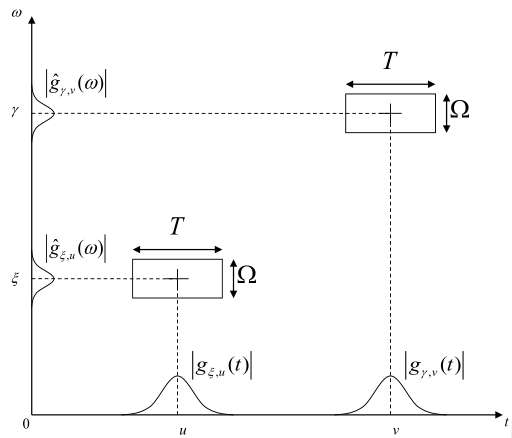2 篇文章 0 订阅

# 如何实现加窗傅里叶变换（WFT）

F(jω)=f(t)ejωtdt

ft(u)=g¯(ut)f(u)

f˜(ω,t)=g¯(ut)f(u)e2πjωudu

# 时频局域化与不确定性定理

t0=1g(t)2t|g(t)|2dt

ω0=1g^(ω)2ω|g^(ω)|2dω

T2=(tt0)2|g(t)|2dt|g(t)|2dt

Ω2=(ωω0)2|g^(ω)|2dω|g^(ω)|2dω# 从“原子”的角度来理解WFT

f˜(ω,t)=g¯(ut)f(u)e2πjωudu

F(jω)=f(t)ejωtdt

gω,t(u)=e2πjωug(ut)

<f,g>=f(t)g¯(t)dt
<script type="math/tex; mode=display" id="MathJax-Element-7436"> = \int^{\infty}_{-\infty}f(t)\bar g(t)dt</script>

|<f,g>|fg

Plancherel定理（其实就是能量守恒定理）：
f=f^

<f,g>=<f^,g^>
<script type="math/tex; mode=display" id="MathJax-Element-7439"> = <\hat f,\hat g></script>

f˜(ω,t)=<f(u),gω,t(u)>

f˜(ω,t)=<f˜,g˜ω,t>

<f˜,g˜ω,t>=f˜(v)g˜¯ω,t(v)dv
<script type="math/tex; mode=display" id="MathJax-Element-7446"><\widetilde f,\widetilde g_{\omega ,t}> = \int^{\infty}_{-\infty}\widetilde f(v)\bar{\widetilde g}_{\omega ,t}(v)dv</script>
=f˜(v)e2πjvtg˜¯ω,t(vω)dv

# 关于高斯窗

1. 它是一种平滑的函数，这相比于一个我们熟悉的矩形窗就要好很多，它能够避免频率域的震荡。
2. 它是一种从零开始平滑到极值再平滑地衰落到0的函数，这符合一个我们常见信号的特点——平滑到增长到极大值然后再平滑地衰落。
3. 它的FT也是一个高斯函数。
4. 它是偶对称的，有很多非常良好的性质。

（注：我所说的常见信号，比如像音乐，或者是图像的亮度信号，他们都有一定的持续期，但是由于惯性，它们都不可能立刻跳到极值（或者稳定值），都有一个充能以及在衰减时候释放能量的过程。选择高斯窗应该说会更好地贴合这个特点。）

08-26
03-011万+02-10
02-061423
05-2783
12-10560
04-214510
12-29
06-092万+
03-1684
03-117297
08-031万+
05-145万+
01-121万+
06-181万+点击重新获取扫码支付余额充值